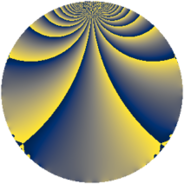# Properties

 Label 1148.1.bjLevel $1148$ Weight $1$ Character orbit 1148.bj Rep. character $\chi_{1148}(319,\cdot)$ Character field $\Q(\zeta_{12})$ Dimension $8$ Newform subspaces $1$ Sturm bound $168$ Trace bound $0$

# Related objects

## Defining parameters

 Level: $$N$$ $$=$$ $$1148 = 2^{2} \cdot 7 \cdot 41$$ Weight: $$k$$ $$=$$ $$1$$ Character orbit: $$[\chi]$$ $$=$$ 1148.bj (of order $$12$$ and degree $$4$$) Character conductor: $$\operatorname{cond}(\chi)$$ $$=$$ $$1148$$ Character field: $$\Q(\zeta_{12})$$ Newform subspaces: $$1$$ Sturm bound: $$168$$ Trace bound: $$0$$

## Dimensions

The following table gives the dimensions of various subspaces of $$M_{1}(1148, [\chi])$$.

Total New Old
Modular forms 24 24 0
Cusp forms 8 8 0
Eisenstein series 16 16 0

The following table gives the dimensions of subspaces with specified projective image type.

$$D_n$$ $$A_4$$ $$S_4$$ $$A_5$$
Dimension 0 0 8 0

## Trace form

 $$8q + 4q^{4} + O(q^{10})$$ $$8q + 4q^{4} - 4q^{10} - 8q^{13} - 4q^{16} + 4q^{26} + 4q^{40} - 4q^{42} - 4q^{52} - 4q^{53} + 8q^{57} - 8q^{64} - 4q^{65} + 4q^{66} - 4q^{81} + 4q^{82} - 4q^{89} - 4q^{93} - 8q^{98} + O(q^{100})$$

## Decomposition of $$S_{1}^{\mathrm{new}}(1148, [\chi])$$ into newform subspaces

Label Dim. $$A$$ Field Image CM RM Traces $q$-expansion
$$a_2$$ $$a_3$$ $$a_5$$ $$a_7$$
1148.1.bj.a $$8$$ $$0.573$$ $$\Q(\zeta_{24})$$ $$S_{4}$$ None None $$0$$ $$0$$ $$0$$ $$0$$ $$q-\zeta_{24}^{2}q^{2}-\zeta_{24}^{7}q^{3}+\zeta_{24}^{4}q^{4}+\cdots$$